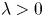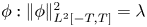Hostname: page-component-546b4f848f-hhr79 Total loading time: 0 Render date: 2023-05-30T12:46:45.542Z Has data issue: false Feature Flags: { "useRatesEcommerce": true } hasContentIssue false

# Stability of periodic waves for the fractional KdV and NLS equations

Published online by Cambridge University Press:  12 August 2020

## Abstract

We consider the focussing fractional periodic Korteweg–deVries (fKdV) and fractional periodic non-linear Schrödinger equations (fNLS) equations, with L2 sub-critical dispersion. In particular, this covers the case of the periodic KdV and Benjamin-Ono models. We construct two parameter family of bell-shaped travelling waves for KdV (standing waves for NLS), which are constrained minimizers of the Hamiltonian. We show in particular that for each$\lambda > 0$, there is a travelling wave solution to fKdV and fNLS$\phi : \|\phi \|_{L^2[-T,T]}^2=\lambda$, which is non-degenerate. We also show that the waves are spectrally stable and orbitally stable, provided the Cauchy problem is locally well-posed in Hα/2[ − T, T] and a natural technical condition. This is done rigorously, without any a priori assumptions on the smoothness of the waves or the Lagrange multipliers.

Type
Research Article
Information

## Access options

Get access to the full version of this content by using one of the access options below. (Log in options will check for institutional or personal access. Content may require purchase if you do not have access.)

## References

Amick, C. J. and Toland, J. F.. Uniqueness and related analytic properties for the Benjamin-Ono equation - a nonlinear Neumann problem in the plane. Acta Math. 167 (1991), 107126.CrossRefGoogle Scholar
Angulo Pava, J., Bona, J. L. and Scialom, M.. Stability of cnoidal waves. Adv. Differential Equations. 11 (2006), 13211374.Google Scholar
Angulo Pava, J.. Nonlinear stability of periodic traveling waves olutions to the Schrödinger and the modified Korteweg-de Vries equations. J. Differential Equ. 235 (2007), 130.CrossRefGoogle Scholar
Angulo Pava, J. and Natali, F.. Positivity properties of the Fourier transform and the stability of periodic travelling-wave solutions. SIAM J. Math. Anal. 40 (2008), 11231151.CrossRefGoogle Scholar
Angulo Pava, J.. Nonlinear dispersive equations. Existence and stability of solitary and periodic travelling wave solutions. In Mathematical surveys and monographs, vol. 156(Providence, RI: American Mathematical Society, 2009).Google Scholar
Angulo Pava, J., Scialom, M. and Banquet, C.. The regularized Benjamin-Ono and BBM equations: Well-posedness and nonlinear stability. J. Differential Equ. 250 (2011), 40114036.CrossRefGoogle Scholar
Benjamin, T. B.. The stability of solitary waves. R. Soc. Lond. Proc. Ser. A Math. Phys. Eng. Sci. 328 (1972), 153183.Google Scholar
Bona, J. L., Souganidis, P. E. and Strauss, W. A.. Stability and instability of solitary waves of Korteweg-de Vries type. R. Soc. Lond. Proc. Ser. A Math. Phys. Eng. Sci. 411 (1987), 395412.Google Scholar
Claasen, K. and Johnson, M.. Nondegeneracy and Stability of Antiperiodic Bound States for Fractional Nonlinear Schrödinger Equations. J. Differential Equ. 266 (2019), 56645712.CrossRefGoogle Scholar
Colliander, J., Keel, M., Staffilani, G., Takaoka, H. and Tao, T.. Sharp global well- posedness for KdV and modified KdV on ℝ and${\mathbb {T}}$. J. Amer. Math. Soc. 16 (2003), 705749.CrossRefGoogle Scholar
Frank, R. and Lenzmann, E.. Uniqueness of non-linear ground states for fractional Laplacians in ℝ. Acta Math. 210 (2013), 261318.Google Scholar
Grillakis, M., Shatah, J. and Strauss, W.. Stability theory of solitary wavs in the presence of symmetry I. J. Funct. Anal. 74 (1987), 160197.CrossRefGoogle Scholar
Herr, S., Ionescu, A., Kenig, C. and Koch, H.. A para-differential renormalization technique for nonlinear dispersive equations. Comm. Partial Differential Equ. 35 (2010), 18271875.CrossRefGoogle Scholar
Hur, V. and Johnson, M.. Stability of periodic traveling waves for nonlinear dispersive equations. SIAM J. Math. Anal. 47 (2015), 35283554.CrossRefGoogle Scholar
Hur, V., Johnson, M. and Martin, J.. Oscillation estimates of eigenfunctions via the combinatorics of non-crossing partitions. Discrete Anal., 2017), Paper No. 13, 20 pp.Google Scholar
Hur, V.. Norm inflation for equations of KdV type with fractional dispersion. Differential Integral Equ. 31 (2018), 833850.Google Scholar
Ionescu, A. and Kenig, C.. Global well-posedness of the Benjamin-Ono equation in low-regularity spaces. J. Amer. Math. Soc. 20 (2007), 753798.CrossRefGoogle Scholar
Kapitula, T. and Promislow, K.. Spectral and dynamical stability of nonlinear waves. In Applied mathematical sciences, vol. 185 ( New York: Springer, 2013).Google Scholar
Kenig, C. and Martel, Y.. Asymptotic stability of solitons for the Benjamin-Ono equation. Rev. Mat. Iberoam. 25 (2009), 909970.CrossRefGoogle Scholar
Kenig, C. E., Martel, Y. and Robbiano, L.. Local well-posedness and blow-up in the energy space for a class of L 2 critical dispersion generalized Benjamin-Ono equations. Ann. Inst. H. Poincaré Anal. Non Linéaire. 28 (2011), 853887.CrossRefGoogle Scholar
Klein, C., Linares, F., Pilod, D. and Saut, J. C.. On Whitham and related equations. Stud. Appl. Math. 140 (2018), 133177.CrossRefGoogle Scholar
Le, U. and Pelinovski, D.. Convergence of Petviashvili’s method near periodic waves of the fractional Korteweg-De Vries equation. SIAM J. Math. Anal. 51 (2019), 28502883.CrossRefGoogle Scholar
Lin, Z. and Zeng, C.. Instability, index theorem, and exponential trichotomy for Linear Hamiltonian PDEs, available at https://arxiv.org/abs/1703.04016, to appear as Mem. Amer. Math. Soc.Google Scholar
Linares, F., Pilod, D. and Saut, J. C.. Remarks on the orbital stability of ground state solutions of fKdV and related equations. Adv. Differential Equ. 20 (2015), 835858.Google Scholar
Martel, Y. and Merle, F.. Asymptotic stability of solitons for subcritical generalized KdV equations. Arch. Ration. Mech. Anal. 157 (2001), 219254.CrossRefGoogle Scholar
Molinet, L.. Global well-posedness in L 2 for the periodic Benjamin-Ono equation. Amer. J. Math. 130 (2008), 635683.CrossRefGoogle Scholar
Molinet, L., Pilod, D. and Vento, S.. On well-posedness for some dispersive perturbations of Burgers equation. Ann. Inst. H. Poincaré Anal. Non Linéaire. 35 (2018), 17191756.CrossRefGoogle Scholar
Natali, F., Le, U. and Pelinovsky, D.. New variational characterization of periodic waves in the fractional Korteweg-de Vries equation, available at arXiv:1907.01412.Google Scholar
Nonnenmacher. Lecture notes, S.. Spectral Theory of self-adjoint operators, June 2017, University of Cardiff.Google Scholar
Stefanov, A.. On the normalized ground states of second order PDE’s with mixed power non-linearities. Comm. Math. Phys. 369 (2019), 929971.CrossRefGoogle Scholar
Vakhitov, N. and Kolokolov, A. A.. Stationary solutions of the wave equation in the medium with nonlinearity saturation. Radiophys. Quantum Electron. 16 (1973), 783789.CrossRefGoogle Scholar